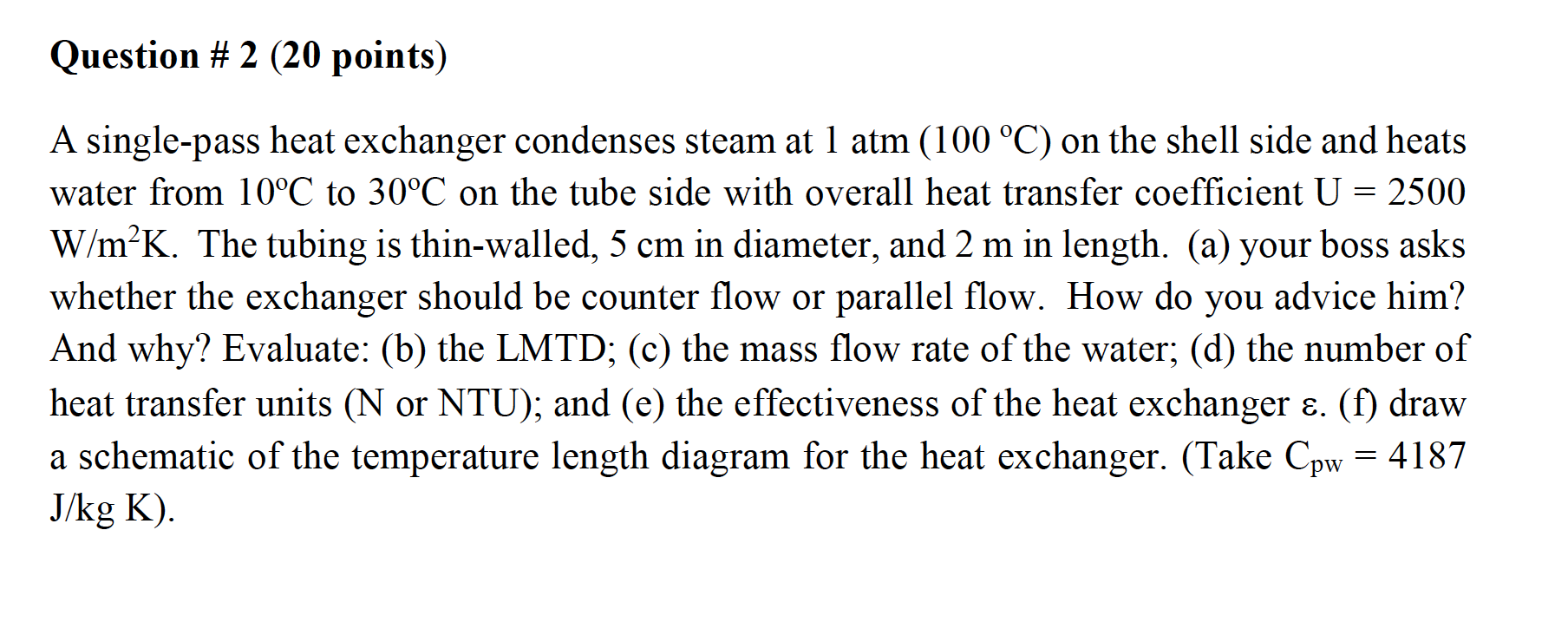# Question Solved1 AnswerQuestion # 2 (20 points) A single-pass heat exchanger condenses steam at 1 atm (100 °C) on the shell side and heats water from 10°C to 30°C on the tube side with overall heat transfer coefficient U = 2500 W/m²K. The tubing is thin-walled, 5 cm in diameter, and 2 m in length. (a) your boss asks whether the exchanger should be counter flow or parallel flow. How do you advice him? And why? Evaluate: (b) the LMTD; (c) the mass flow rate of the water; (d) the number of heat transfer units (N or NTU); and (e) the effectiveness of the heat exchanger ε. (f) draw a schematic of the temperature length diagram for the heat exchanger. (Take Cpw = 4187 J/kg K).9SULQF The Asker · Mechanical EngineeringTranscribed Image Text: Question # 2 (20 points) A single-pass heat exchanger condenses steam at 1 atm (100 °C) on the shell side and heats water from 10°C to 30°C on the tube side with overall heat transfer coefficient U = 2500 W/m²K. The tubing is thin-walled, 5 cm in diameter, and 2 m in length. (a) your boss asks whether the exchanger should be counter flow or parallel flow. How do you advice him? And why? Evaluate: (b) the LMTD; (c) the mass flow rate of the water; (d) the number of heat transfer units (N or NTU); and (e) the effectiveness of the heat exchanger ε. (f) draw a schematic of the temperature length diagram for the heat exchanger. (Take Cpw = 4187 J/kg K).
More
Transcribed Image Text: Question # 2 (20 points) A single-pass heat exchanger condenses steam at 1 atm (100 °C) on the shell side and heats water from 10°C to 30°C on the tube side with overall heat transfer coefficient U = 2500 W/m²K. The tubing is thin-walled, 5 cm in diameter, and 2 m in length. (a) your boss asks whether the exchanger should be counter flow or parallel flow. How do you advice him? And why? Evaluate: (b) the LMTD; (c) the mass flow rate of the water; (d) the number of heat transfer units (N or NTU); and (e) the effectiveness of the heat exchanger ε. (f) draw a schematic of the temperature length diagram for the heat exchanger. (Take Cpw = 4187 J/kg K).